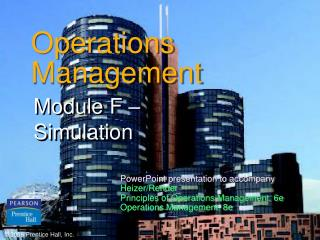DownloadDownload PresentationOperations Management

# Operations Management

Download Presentation## Operations Management

- - - - - - - - - - - - - - - - - - - - - - - - - - - E N D - - - - - - - - - - - - - - - - - - - - - - - - - - -
##### Presentation Transcript

1. Operations Management Module F – Simulation PowerPoint presentation to accompany Heizer/Render Principles of Operations Management, 6e Operations Management, 8e © 2006 Prentice Hall, Inc.

2. What is Simulation? • An attempt to duplicate the features, appearance, and characteristics of a real system • To imitate a real-world situation mathematically • To study its properties and operating characteristics • To draw conclusions and make action decisions based on the results of the simulation

3. Simulation Applications Table F.1

4. Probability of Demand Table F.2

5. Assignment of Random Numbers Table F.3

6. Table of Random Numbers Table F.4

7. Simulation Example 1 Select random numbers from Table F.3

8. 5 i=1 = ∑ (probability of i units) x (demand of i units) = (.05)(0) + (.10)(1) + (.20)(2) + (.30)(3) + (.20)(4) + (.15)(5) = 0 + .1 + .4 + .9 + .8 + .75 = 2.95 tires Expecteddemand Simulation Example 1

9. Advantages of Simulation Relatively straightforward and flexible Can be used to analyze large and complex real-world situations that cannot be solved by conventional models Real-world complications can be included that most OM models cannot permit “Time compression” is possible

10. Advantages of Simulation Allows “what-if” types of questions Does not interfere with real-world systems Can study the interactive effects of individual components or variables in order to determine which ones are important

11. Disadvantages of Simulation Can be very expensive and may take months to develop It is a trial-and-error approach that may produce different solutions in repeated runs Managers must generate all of the conditions and constraints for solutions they want to examine Each simulation model is unique

12. Queuing Simulation Overnight barge arrival rates Table F.5

13. Queuing Simulation

14. Average number of barges delayed to the next day 20 delays 15 days = = 1.33 barges delayed per day = = 2.73 arrivals per night 41 arrivals 15 days Average number of nightly arrivals 39 unloadings 15 days = = 2.60 unloadings per day Average number of barges unloaded each day Queuing Simulation

15. Inventory Simulation Daily demand for Ace Drill Table F.8

16. Inventory Simulation Reorder lead time Table F.9

17. Inventory Simulation Begin each simulation day by checking to see if ordered inventory has arrived. If if has, increase current inventory by the quantity ordered. Generate daily demand using probability distribution and random numbers. Compute ending inventory. If on-hand is insufficient to meet demand, satisfy as much as possible and note lost sales. Determine whether the day's ending inventory has reached the reorder point. If it has, and there are no outstanding orders, place an order. Choose lead time using probability distribution and random numbers.

18. Inventory Simulation Order quantity = 10 units Reorder point = 5 units Table F.10

19. 41 total units 10 days 2 sales lost 10 days Average ending inventory = = 4.1 units/day Average lost sales = = .2 unit/day 3 orders 10 days Average number of orders placed = = .3 order/day Inventory Simulation

20. Inventory Simulation Daily order cost = (cost of placing 1 order) x (number of orders placed per day) = \$10 per orderx .3 order per day = \$3 Daily holding cost = (cost of holding 1 unit for 1 day) x (average ending inventory) = 50¢ per unit per dayx 4.1 units per day = \$2.05 Daily stockout cost = (cost per lost sale) x (averagenumber of lost sales per day) = \$8 per lost salex .2 lost sales per day = \$1.60 Total daily inventory cost = Dailyorder cost + Daily holding cost + Daily stockout cost = \$6.65

21. Using Software in Simulation • Computers are critical in simulating complex tasks • General-purpose languages - BASIC, C++ • Special-purpose simulation languages - GPSS, SIMSCRIPT • Require less programming time for large simulations • Usually more efficient and easier to check for errors • Random-number generators are built in

22. Using Software in Simulation • Commercial simulation programs are available for many applications - Extend, Modsim, Witness, MAP/1, Enterprise Dynamic, Simfactory, ProModel, Micro Saint, ARENA • Spreadsheets such as Excel can be used to develop some simulations